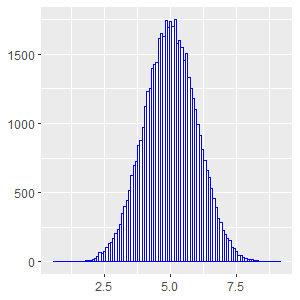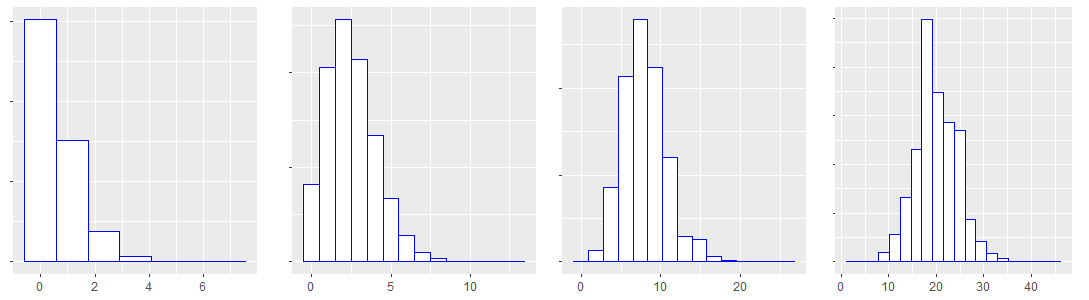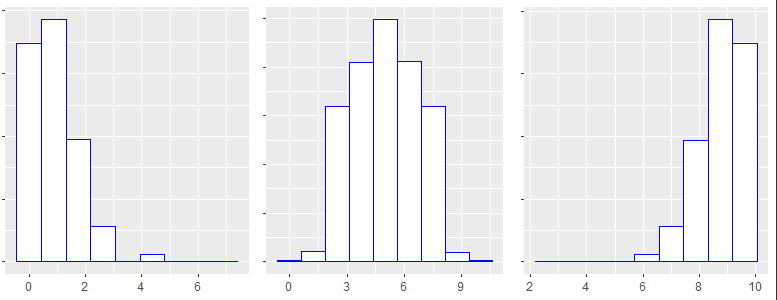# Finding statistical models for analyzing your data

### Foreword

Just so that we are clear about it: searching for which statistical model to use or which statistical test to perform on your data is NOT something to do once you have gathered your data, but it is something that is part of the design of your experiment to come! That being said, it is unfortunately common knowledge that the quest for the right model/test often starts at the last moment... when results need to be interpreted.

The content of this page is based on the assumption that you are in the design phase of the study. Nonetheless, you will find it useful also if you are looking for help when analysing an existing data set.

This key will help you define which model or family of models you may use to analyze your data. For using this tool, you have to know whether your data is clustered or not, which distribution describes it, which type(s) of variables are present. You will find more info about variables, data clustering, distribution and hypothesis formulation further below.

Univariate analysis refers to the simplest form of statistical analysis where a single response variable is considered. In this type of analysis, you investigate whether that single response variable is affected by one or several predictor variables.

Choose now between gaussian/normal and non-normal distribution.

Choose now between Data Clustering and No Data Clustering.

It is recommended that you proceed with a Linear Model (LM).

It is recommended that you proceed with a Linear Mixed-Effects Model (LME).

Choose now between Data Clustering and No Data Clustering.

It is recommended that you proceed with a Generalized Linear Model (GLM).

It is recommended that you proceed with a Generalized Linear Mixed Model (GLMM).

### Formulate a Hypothesis

The first and possibly most important question when planning an experiment with subsequent data analysis is "what is the working hypothesis?". Often, the experimenter formulates the hypothesis that there exists a difference, a relationship or a correlation between two or more groups based on at least one variable. It is this hypothesis (called H1) which will determine the design of the experiment, the population(s) to study, the groups or treatments, the variables, etc. If the hypothesis is not formulated correctly or unclear, chances are great that the experimental design (and data collection) will be sub-optimal, or even inadequate, and that parts of the study (at best) will be discarded. Hence our strong advice: clearly state your hypothesis! Once you have done it, you "logically" have the null hypothesis H0, i.e. the hypothesis that the difference, relationship or correlation that you "hope for" does not exist. And this is that null hypothesis H0 that most statistical tests take as a starting point.

Example1:

• H1: the average size of Benthosema glaciale is different in Masfjorden and Lustrafjorden
• H0: the average size of Benthosema glaciale is NOT different in Masfjorden and Lustrafjorden

Example2:

• H1: the reproductive success of the bean beetle Callosobruchus maculatus increases with temperature.
• H0: the reproductive success of the bean beetle Callosobruchus maculatus does NOT increase with temperature.

Note that, in this case, the experimenter investigates the impact of temperature on the reproductive success of the bean beetle. The formulation of H1 is particular since it states that the effect is limited to an increase, not a difference (decrease AND increase). Thus, the experimenter must reject H1 and accept H0 if the results show either a decrease, or an absence of effect.

It is essential to understand what type of variable(s) you are dealing with and which use you will make of it before starting the analysis. Variable types are numerous: predictor, response, continuous, ordinal, dependent, independent, continuous, etc. and some of these terms are synonymous.

The choice of a statistical test or model depends on several factors, one of which being the type of variable that defines your predictor and response variables. Variables may be split into two categories: categorical or continuous. Note that the variable type is sometimes tricky to determine...

Categorical variables are variables which have only a limited/finite number of values. They are often called discrete or discontinuous variables. All qualitative variables are categorical. There are several types of categorical variables: nominal, ordinal/ranked, dichotomous or binary.

### Nominal variables

A nominal variable has two or more categories or levels. These levels cannot be ranked, nor do they follow a specific order.

Example 1: "blue", "red" and "yellow" are the three levels of the variable "primary color".
Example 2: "Oslo", "Bergen", "Trondheim", "Stavanger" are four levels of the variable "Norwegian cities".

### Ordinal/Ranked variables

A ranked or ordinal variable is a nominal variable which levels can be ordered or ranked.

Example 1: "Low", "Medium" and "High" are three ranked levels of the variable "Intensity".
Example 2: "A", "B", "C", "D", "E" and "F" are six ranked levels of "Grade".
Example 3: "Monday", "Tuesday", "Wednesday", "Thursday", "Friday, "Saturday" and "Sunday" are the time-ordered levels of the variable "Weekdays".

### Dichotomous/Binary variables

A dichotomous variable is a variable that can only take 2 values. A binary variable is a specific type of dichotomous variable where the values taken are either 0 or 1.

Example 1: "TRUE" vs. "FALSE".
Example 2: "Passed" vs. "Failed".
Example 3: "0" vs. "1".

In statistical models, we may differentiate variables based on the way we use them. Both categorical and continuous variables may thus serve as response or predictor variable. The response variable is the variable that changes as a function of the predictor variable(s).

Predictor variables are also called controlled, manipulated, independent or input variables. Response variables are also called measured, explained, experimental, dependent or output variables.

Example: a study aims at evaluating the effect of different food diets om fish growth and measures bodyweight at different timepoints during the season. Here, both time and diet are predictor variables, while bodyweight is the only response variable.

### Data Clustering/Dependency

Before starting the analysis, you must determine whether there may exist a form of dependency or clustering in your data, meaning that the values of some observations are dependent due to, for example, experimental design or sampling conditions. Here, the question is whether there is a clustering factor that may be responsible for coordinated variation of a measured variable(s) in several observations. Remember that this is of great importance when choosing a model for your analysis, since some models assume that observations are independent, while others accept dependency.

Nested design is a typical example of clustering. Let's consider a study where the number of eggs laid by 10 female hawks is measured at three different locations during a unique season. Each hawk is found at a specific location and it is plausible that most, if not all hawks from a single location are affected by local conditions (food availability, human presence, etc) which may have an effect on the reproductive capacity of those birds. Thus each location is a cluster and observations within each cluster must be considered as dependent.

Repeated measurement is also a typical example of clustering. Let's consider a study involving several rats which bodyweight is measured at regular intervals. Since individual rats might have different growth rates for various reasons (dominant behavior, health conditions, etc), all measurements specific to one individual are dependent and the individual itself is the cluster.

### Determine the Data Distribution

Another essential feature of your data that is crucial when choosing a statistical model or test is its distribution. Often, you will see that the data follows a normal/gaussian. Non-normal distributions include poisson or binomial distribution. Here is more info about them.Normal distribution, also called Gaussian- or bell-shaped distribution, is certainly the most common distribution in statistics. Normal distributions are related to continuous variables, and have no bounds (they may take any value between -∞ and +∞).

Several of the most frequently used statistical tests such as Student's t-test and ANOVA rely on the assumption that the data is normally distributed. The Shapiro-Wilks test is a common test for normality of a sample. A Q-Q plot may also be used to visually assess distribution normality.

Note that the shape of distribution does not change, unlike Poisson and binomial distributions.

A Poisson distribution is encountered when handling discrete numerical variables such as count variables (i.e. variables that describe the frequency/occurrence of an event). Count variables are discrete variables bounded at 0, meaning that they may take any countable value between 0 and +∞, but cannot be negative.

The shape of a Poisson distribution is not fixed, but varies with the sample mean, unlike the normal distribution which remains symmetric regardless of the sample mean). It may be skewed when the mean is low, highly-skewed when the mean is close to zero, or even close to the shape of a normal distribution when the mean is large enough.Binomial distribution are often linked to experiments consisting of a finite number of trials for which the outcome is either success or failure, and where the probability p of success/failure is determined. The binomial distribution reflects the probability of reaching a goal after N trials for a given success probability.

As for a Poisson distribution, a binomial distribution describes the behavior of a discrete variable, but it has both zero as a lower bound and an upper bound (finite number of trials). The shape of a binomial distribution, unlike a normal distribution, may vary.